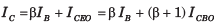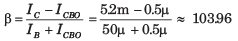Courses

# The Bipolar Junction Transistor - MCQ Test

## 20 Questions MCQ Test GATE ECE (Electronics) 2022 Mock Test Series | The Bipolar Junction Transistor - MCQ Test

Description
This mock test of The Bipolar Junction Transistor - MCQ Test for Electronics and Communication Engineering (ECE) helps you for every Electronics and Communication Engineering (ECE) entrance exam. This contains 20 Multiple Choice Questions for Electronics and Communication Engineering (ECE) The Bipolar Junction Transistor - MCQ Test (mcq) to study with solutions a complete question bank. The solved questions answers in this The Bipolar Junction Transistor - MCQ Test quiz give you a good mix of easy questions and tough questions. Electronics and Communication Engineering (ECE) students definitely take this The Bipolar Junction Transistor - MCQ Test exercise for a better result in the exam. You can find other The Bipolar Junction Transistor - MCQ Test extra questions, long questions & short questions for Electronics and Communication Engineering (ECE) on EduRev as well by searching above.
QUESTION: 1

### A uniformly doped silicon pnp transistor is biased in the forward-active mode. The doping profile is NE = 108 cm-3, NB = 5.2 x 1016 cm-3 and NC = 1015  cm-3 .For VEB = 0.6 V, the pB at x =0 is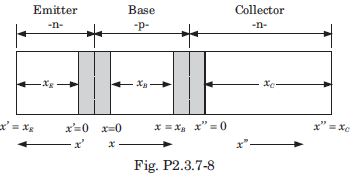Solution: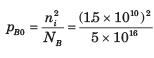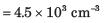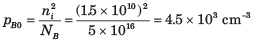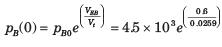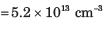QUESTION: 2

### An npn bipolar transistor having uniform doping of NE = 1018 cm-3  NB = 1016 cm-3 and Nc = 6 x 1015 cm-3 is operating in the inverse-active mode with VBE = - 2 V and VBC = 0.6 V. The geometry of transistor is shown in fig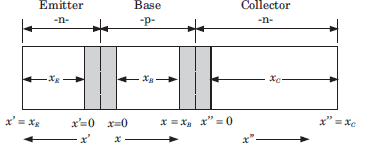Q. The minority carrier concentration at x =  xB is

Solution: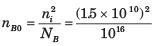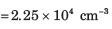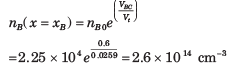QUESTION: 3

### An npn bipolar transistor having uniform doping of NE = 1018 cm-3  NB = 1016 cm-3 and Nc = 6 x 1015 cm-3 is operating in the inverse-active mode with VBE = - 2 V and VBC = 0.6 V. The geometry of transistor is shown in figQ. The minority carrier concentration at x" = 0 is

Solution: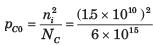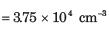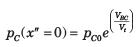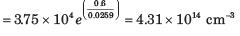QUESTION: 4

An pnp bipolar transistor has uniform doping of NE = 6 x 1017 cm-3, N= 2 x 1016  cm-3 and N= 5 x 1014 cm-3. The transistor is operating is inverse-active mode. The maximum VCB voltage, so that the low injection condition applies, is

Solution:

Low injection limit is reached when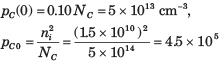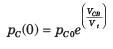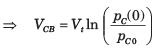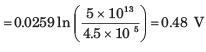QUESTION: 5

The following currents are measured in a uniformly doped npn bipolar transistor:

InE  = 1.20 mA, IpE  = 0.10 mA, InC = 1.18 mA
IR = 0.20 mA, IG = 1 μA, IpC0 = 1 μA

Q.

The α is

Solution: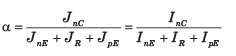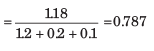QUESTION: 6

The following currents are measured in a uniformly doped npn bipolar transistor:

InE  = 1.20 mA, IpE  = 0.10 mA, InC = 1.18 mA
IR = 0.20 mA, IG = 1 μA, IpC0 = 1 μA

Q.

The β is

Solution: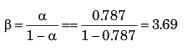QUESTION: 7

The following currents are measured in a uniformly doped npn bipolar transistor:

InE  = 1.20 mA, IpE  = 0.10 mA, InC = 1.18 mA
IR = 0.20 mA, IG = 1 μA, IpC0 = 1 μA

Q.

The  γ is

Solution: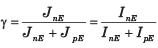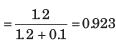QUESTION: 8

A silicon npn bipolar transistor has doping concentration of NE = 2 x 1018cm-3, NB =1017cm-3 and N = 15 x 1016 cm-3. The area is 10-3  cm2 and neutral base width is 1 μm. The transistor is biased in the active region at VBE = 0.5 V. The collector current is
(DB = 20 cm2/s)

Solution: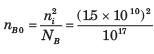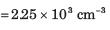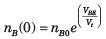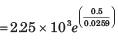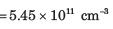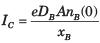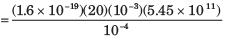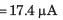QUESTION: 9

A uniformly doped npn bipolar transistor has following parameters:

NE = 1018 cm-3 NB = 5 x 1016 cm-3,
Nc = 2 x 1019 cm-3,
DE = 8 cm2 /s , DB = 15 cm2 /s , Dc = 14 cm2 /s
xE = 0.8 μm, xB = 0.7 μm

The emitter injection efficiency γ is

Solution: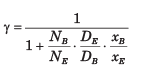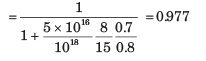QUESTION: 10

In bipolar transistor biased in the forward-active region the base current is IB = 50 μA. and the collector currents is IC = 27 μA. The α is

Solution: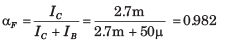QUESTION: 11

For the transistor in fig., IS = 1015 A, βF = 100,  βR = 1. The current ICBO is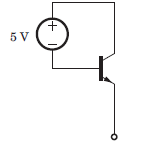Solution: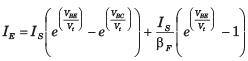= 0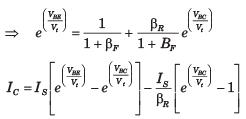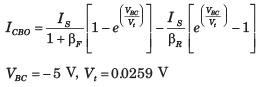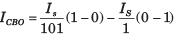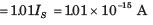QUESTION: 12

Determine the region of operation for the transistor shown in circuit in question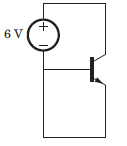Solution: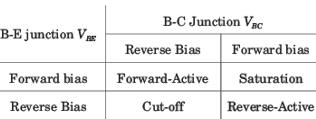VBE = 0 , VBC < 0, Thus both junction are in reverse bias. Hence cut off region.

QUESTION: 13

Determine the region of operation for the transistor shown in circuit in question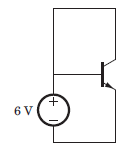Solution:

VBE > 0, VBC = 0, Base-Emitter junction forward bais, Base-collector junction reverse bias, Hence forward-active region.

QUESTION: 14

Determine the region of operation for the transistor shown in circuit in question.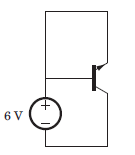Solution:

VBE = 0, VBC> 0, Base-Emitter junction reverse bais , Base-collector junction forward bias, Hence reverse-active region.

QUESTION: 15

Determine the region of operation for the transistor shown in circuit in question.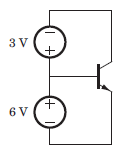Solution:

VBE = 6V, VBC  = 3V Both junction are forward biase, Hence saturation region.

QUESTION: 16

For the circuit shown in fig., let the value of βR =0.5 and  βF = 50. The saturation current is 10-16 A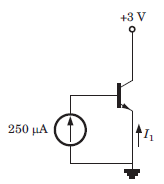Q. The base-emitter voltage is

Solution:

The current source will forward bias the base-emitter junction and the collector base junction will then be reverse biased. Therefore the transistor is in the forward active region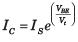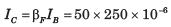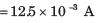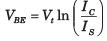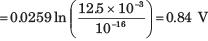QUESTION: 17

For the circuit shown in fig., let the value of βR =0.5 and  βF = 50. The saturation current is 10-16 AQ. The current I1 is

Solution: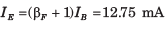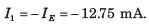QUESTION: 18

The leakage current of a transistor are ICBO = 5μA and ICEO = 0.4 mA, and IB =30 μA

Q. The value of β is

Solution: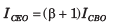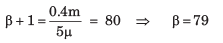QUESTION: 19

The leakage current of a transistor are ICBO = 5μA and ICEO = 0.4 mA, and IB =30 μA

Q. The value of IC is

Solution:

Ic = βIB + ICEO = 79(30μ) + 0.4m = 2.77 mA

QUESTION: 20

For a BJT, IC = 5 mA, IB = 50 μA and ICBO = 0.5μA.

Q. The value of β is

Solution: